# RD Sharma Solutions for Class 12 Maths Chapter 13 Derivatives as a Rate Measurer

## RD Sharma Solutions for Class 12 Maths Chapter 13 – Free PDF Download Updated for (2021-22)

RD Sharma Solutions for Class 12 Chapter 13 – Derivatives as a Rate Measurer provided here. These solutions are in a detailed approach, which helps reassure students and makes the exam preparation effective. Practice is essential during preparations to score well in Mathematics. Hence, we at BYJU’S have provided RD Sharma Solutions in a simple and lucid manner as per the student’s understanding capacity. RD Sharma Solutions can boost the students to perform very well in their upcoming board exams.

Students can access RD Sharma Solutions for Class 12 Maths Chapter 13 Derivatives as a Rate Measurer from the links below. This chapter of RD Sharma Solutions for Class 12 mainly focused on the instantaneous rate of change of a given physical quantity. These solutions are formulated based on the latest CBSE board. Some of the essential topics of this chapter are listed below.

• Definition of derivative as a rate measurer
• Meaning of derivative as a rate measurer
• Theorems and remarks on derivative as a rate measurer
• Definition and meaning of related rates

## RD Sharma Solutions Class 12 Maths Chapter 13 Derivatives as a Rate Measurer: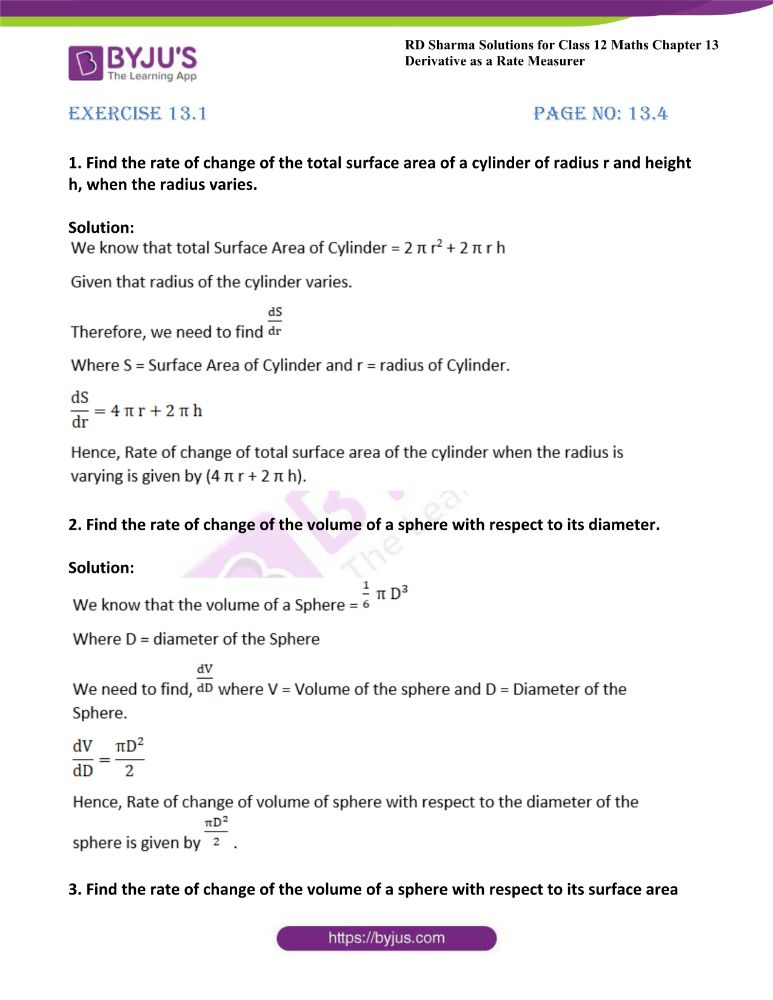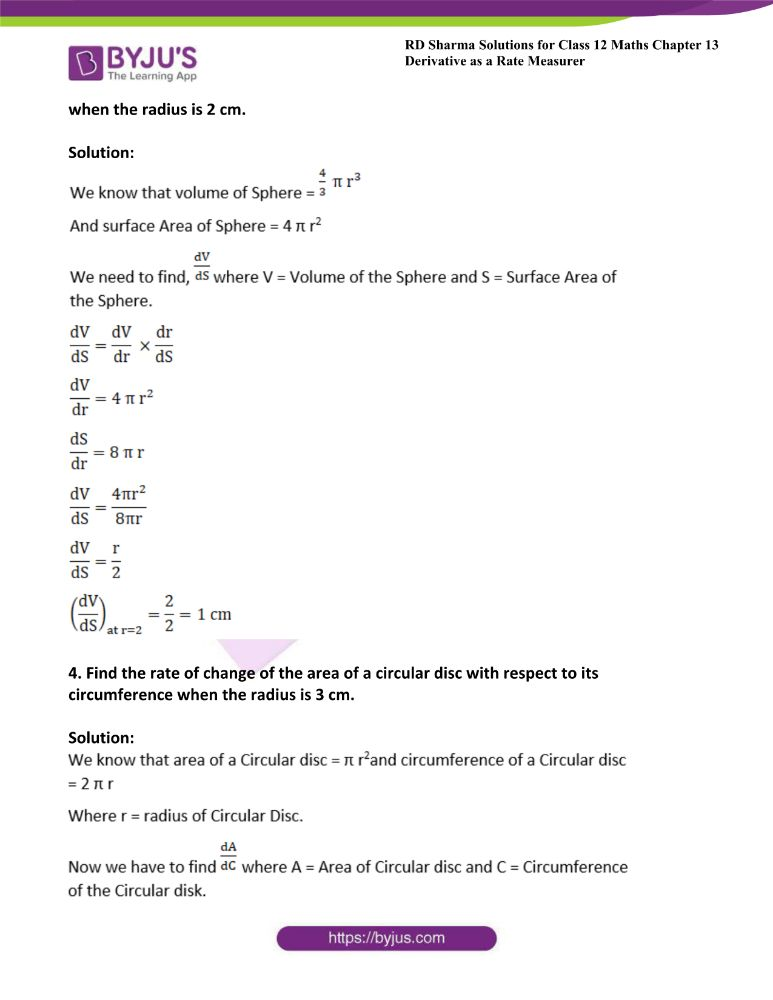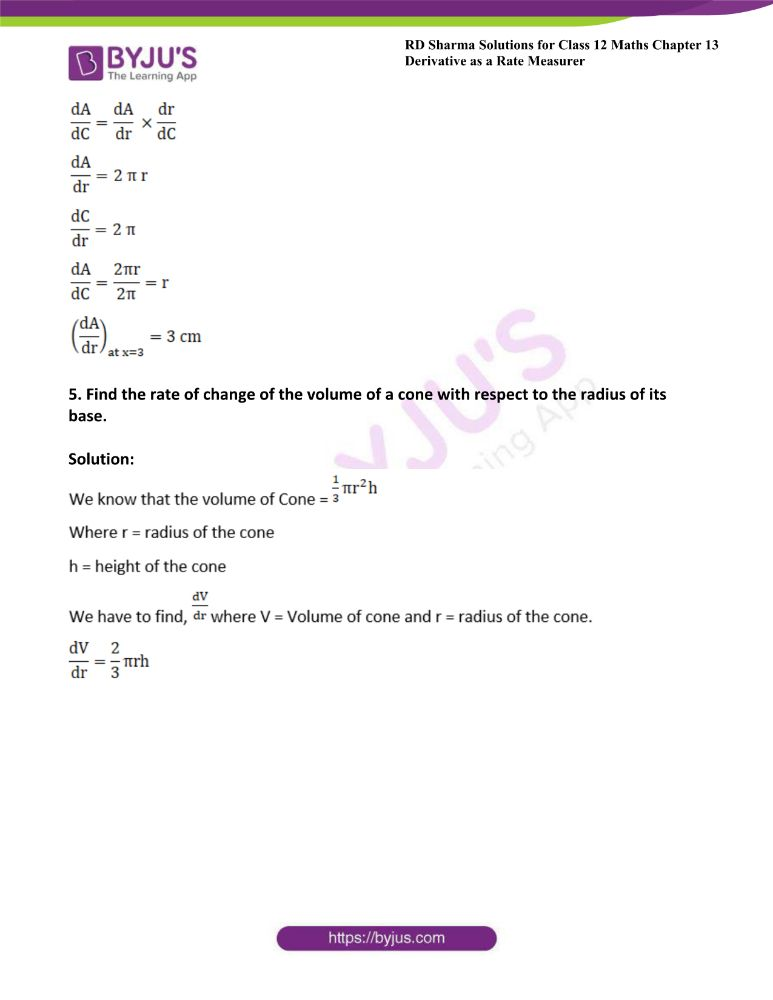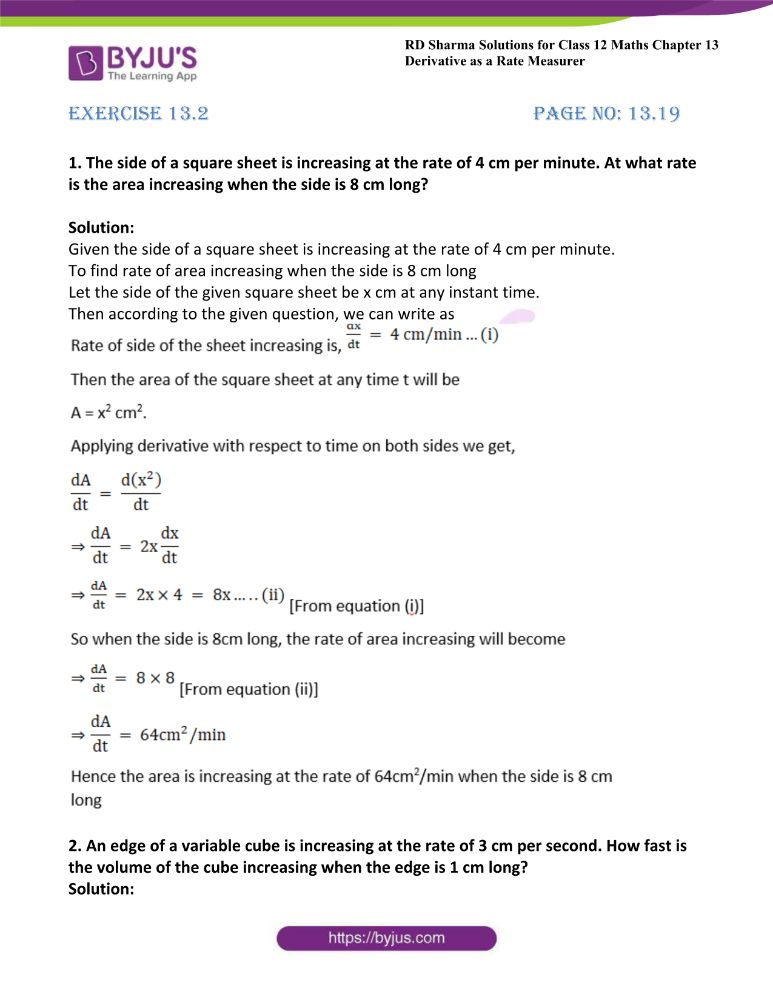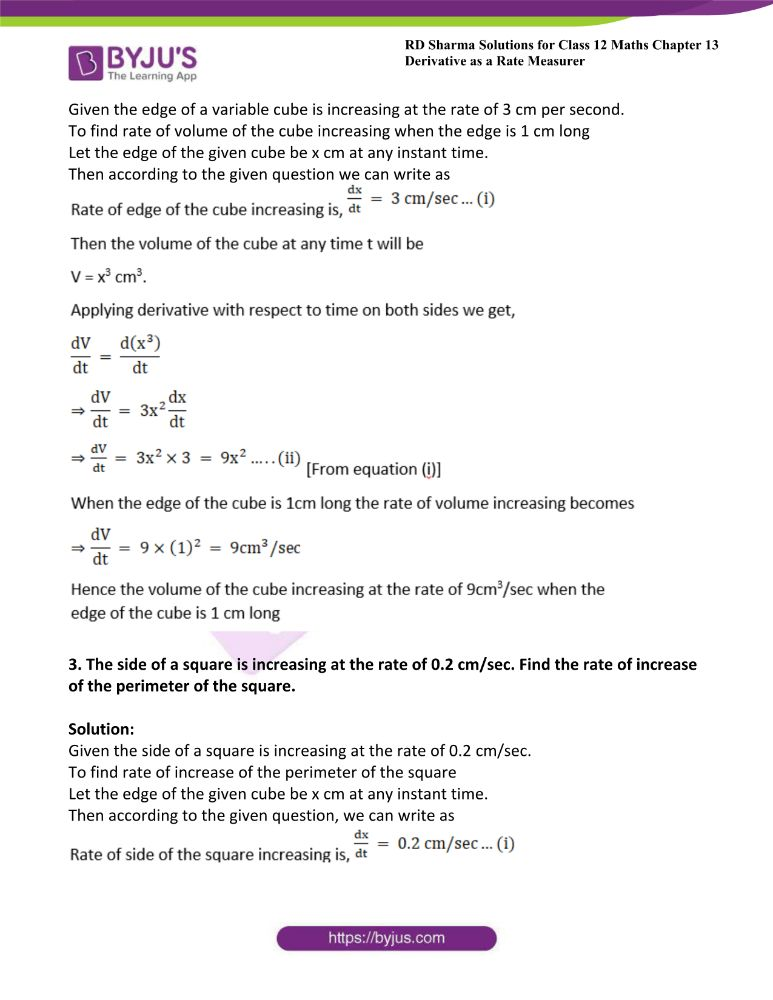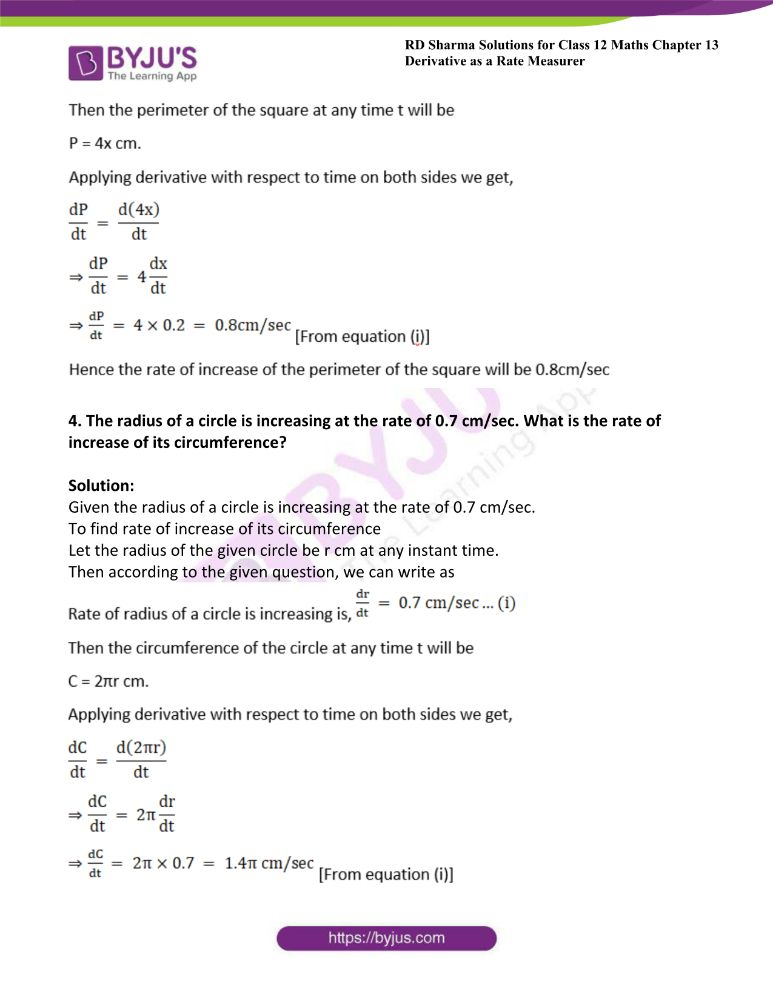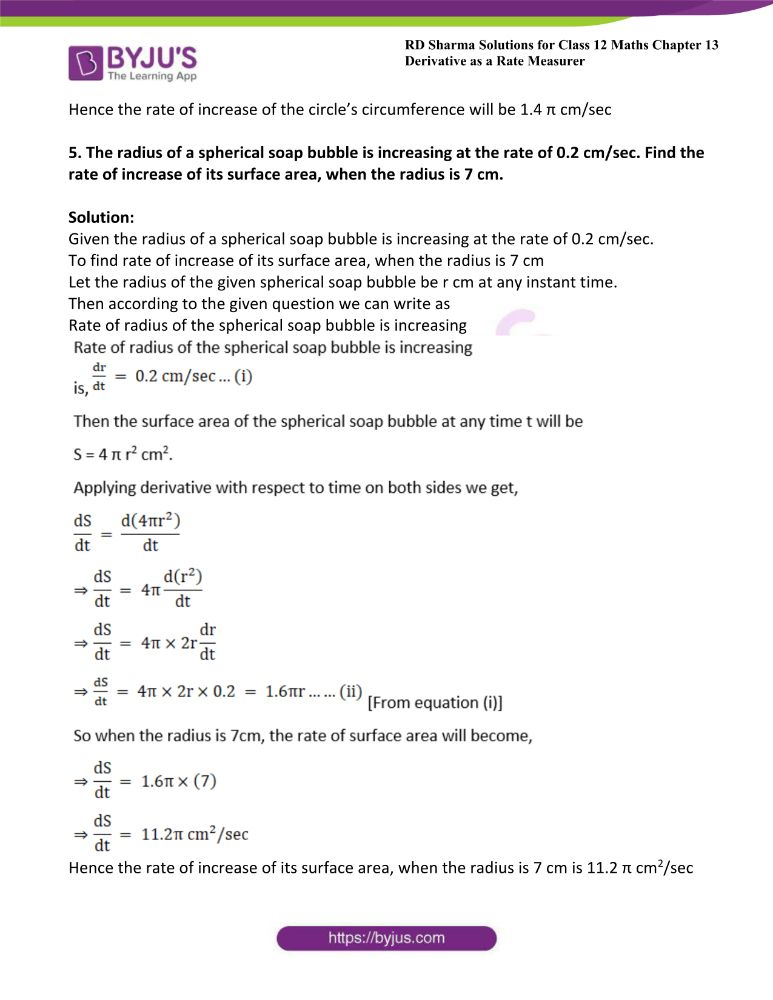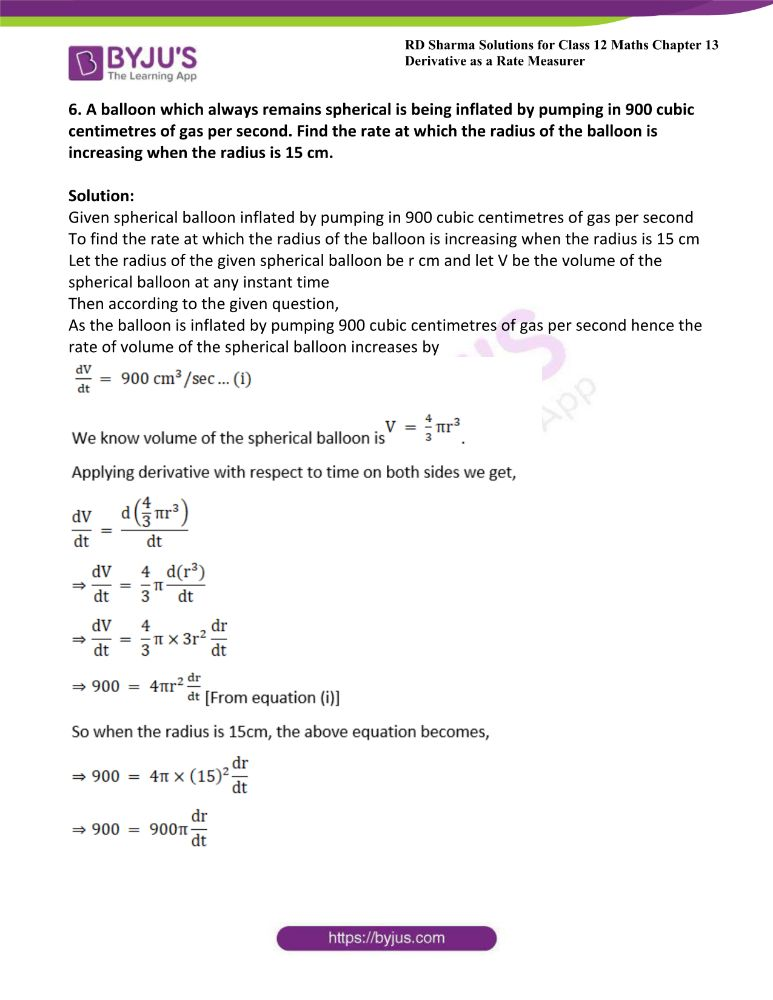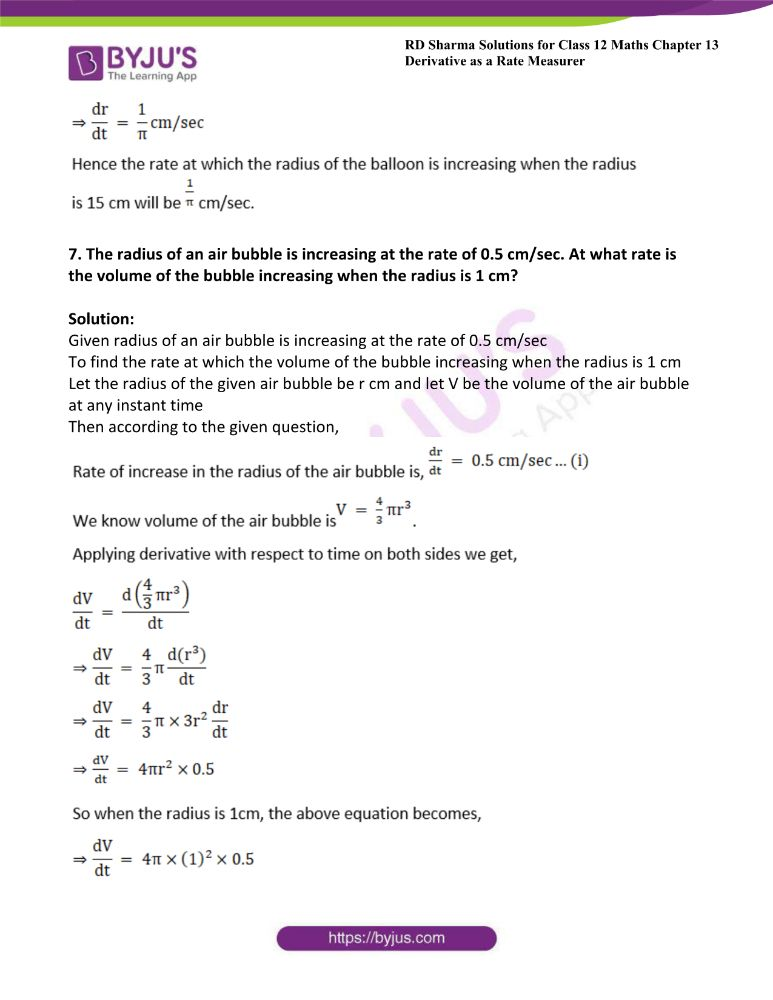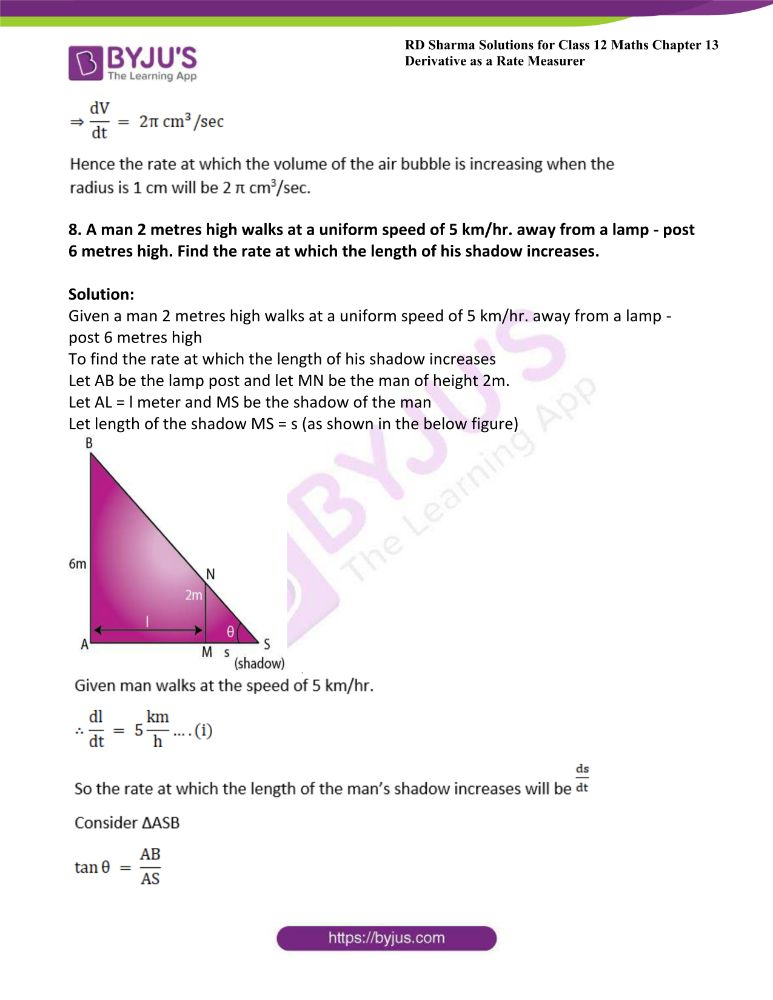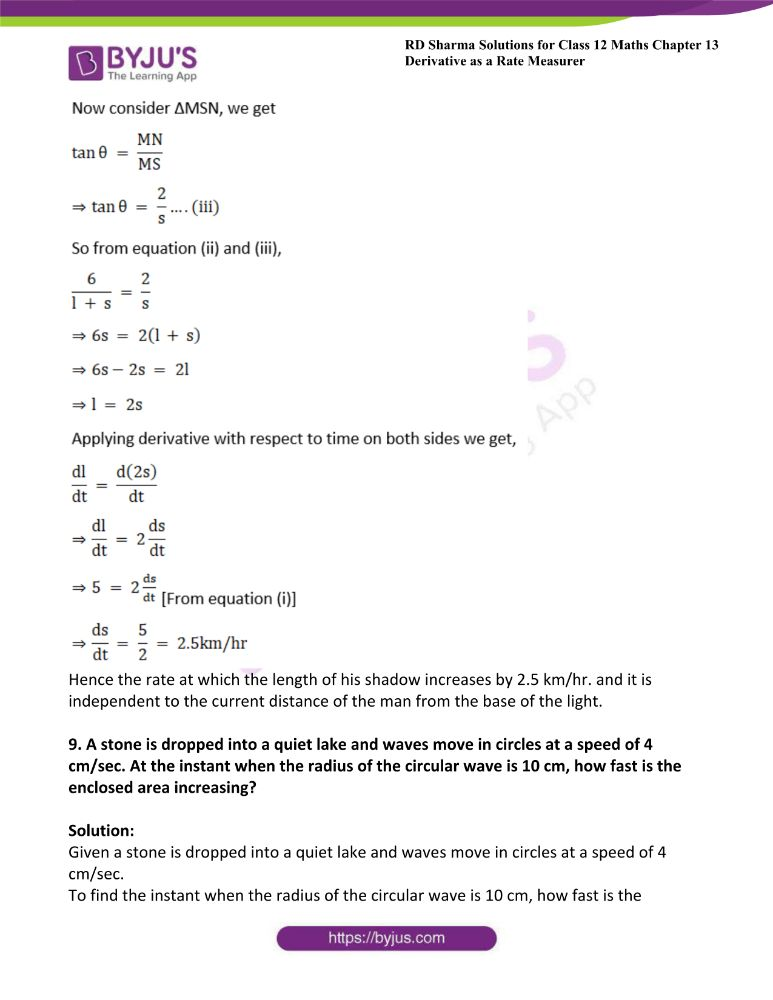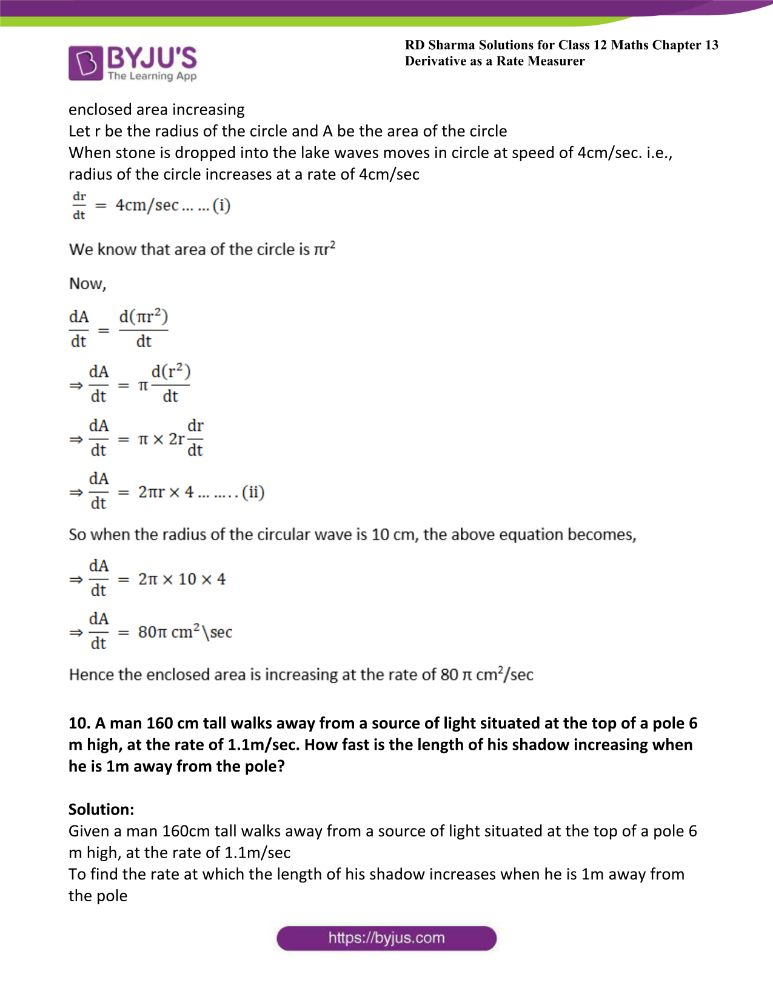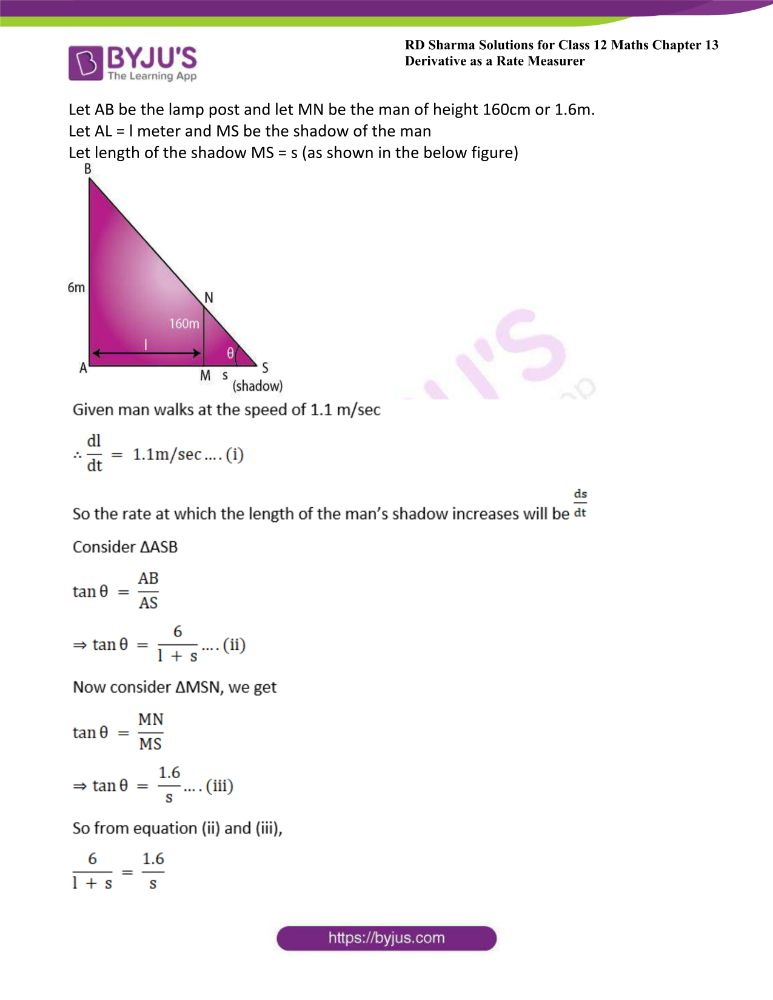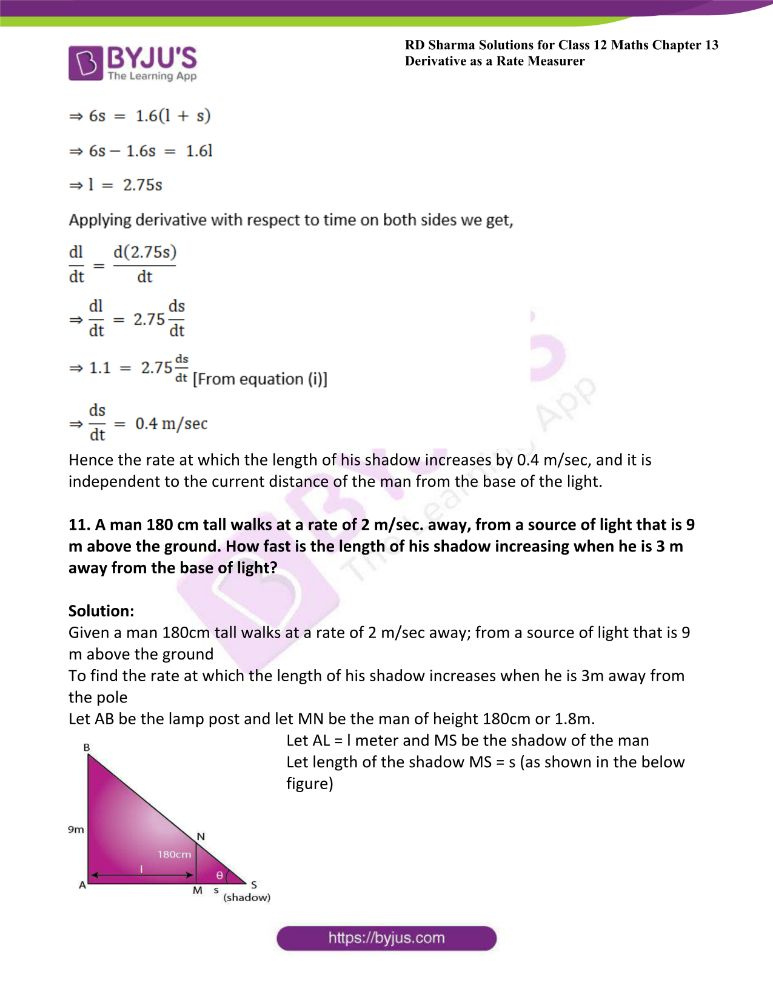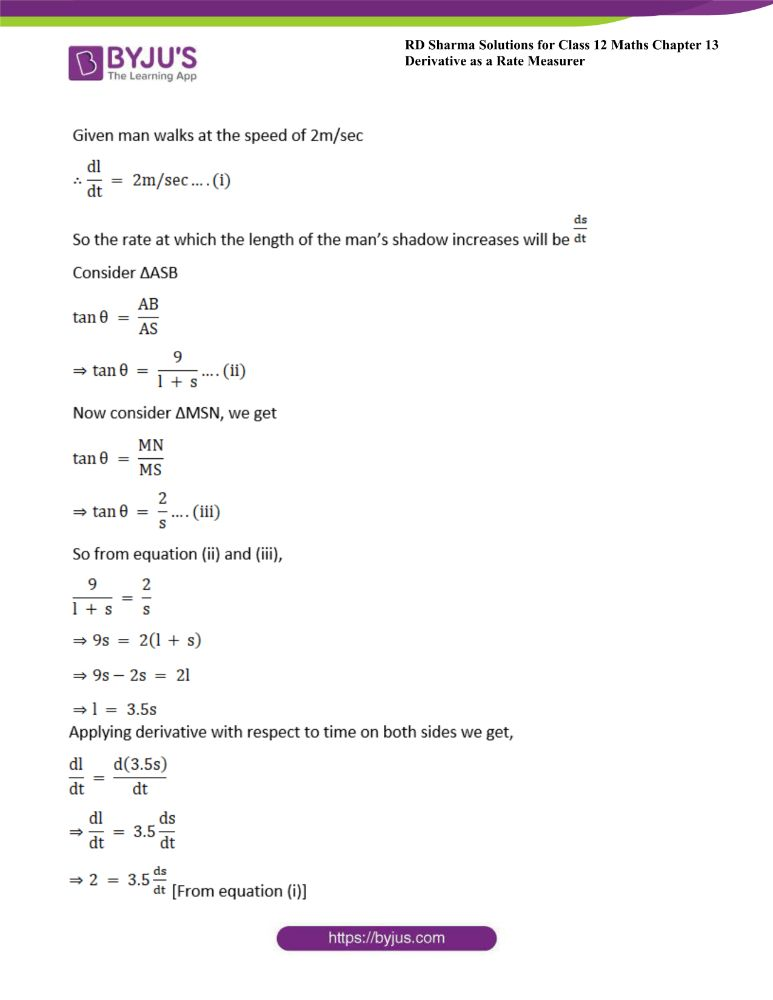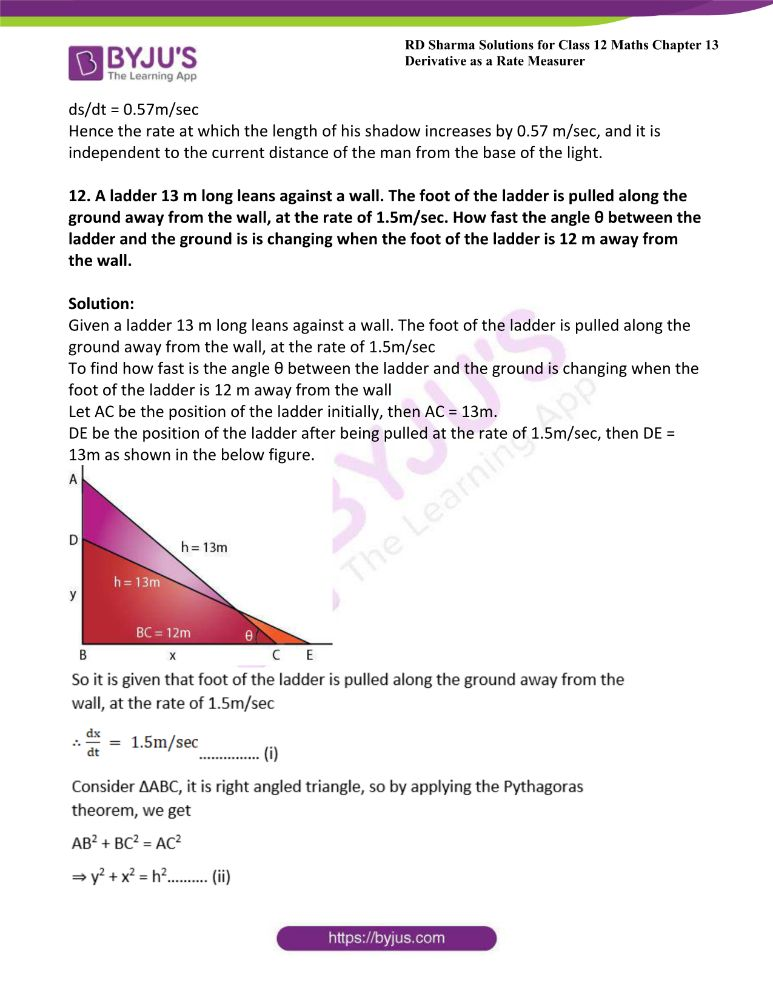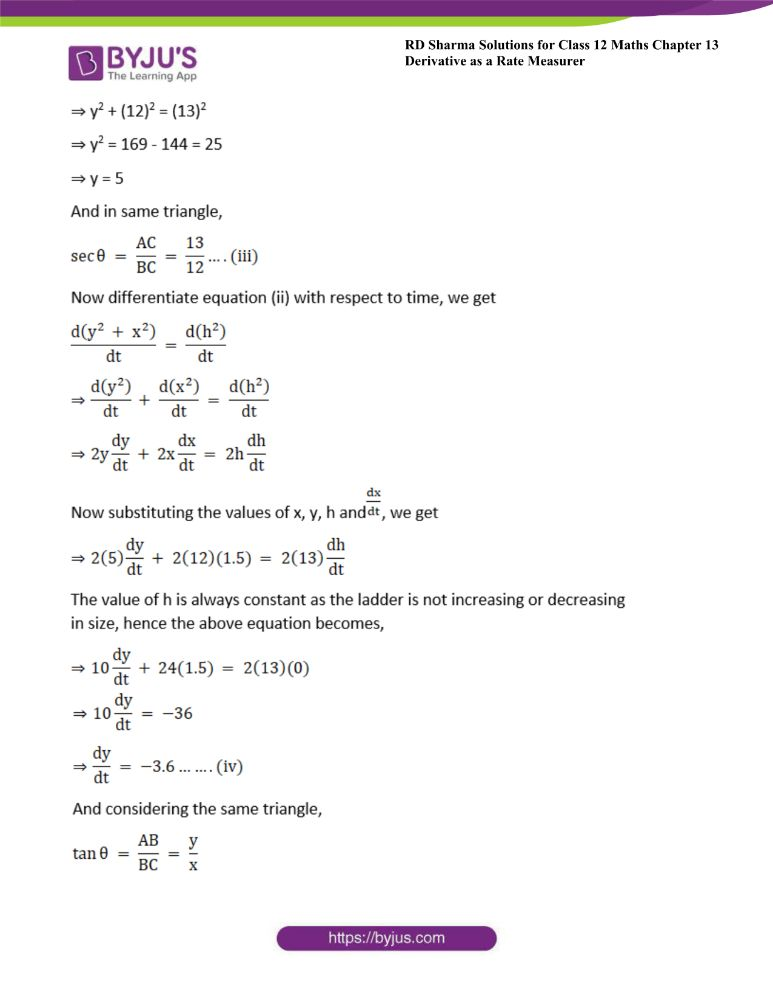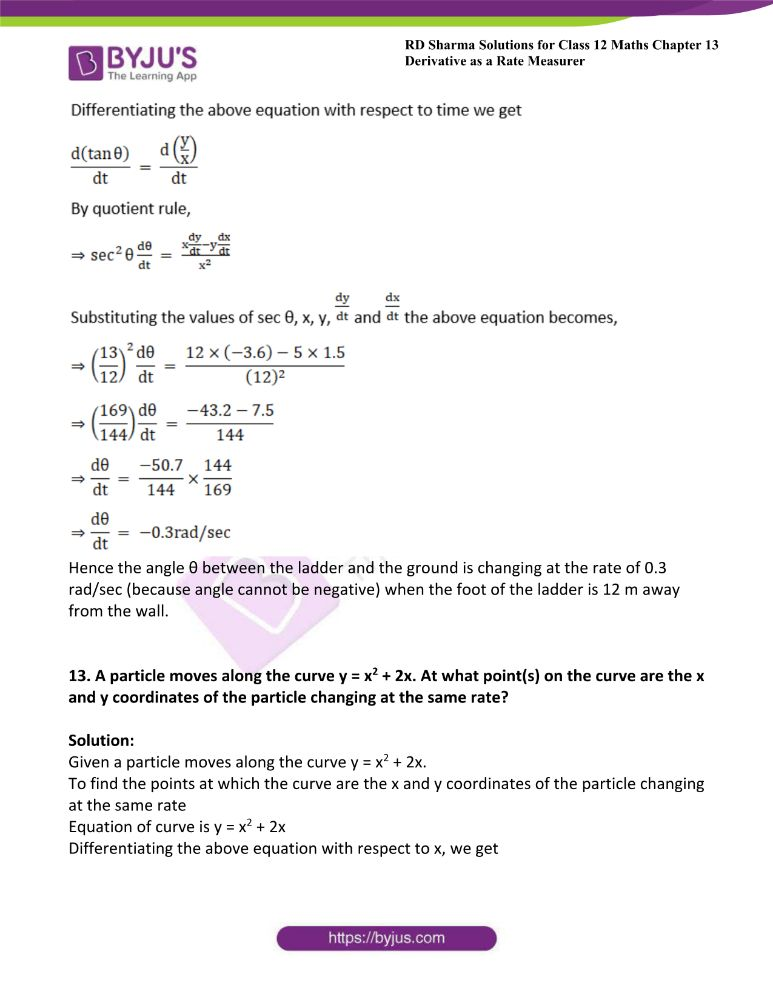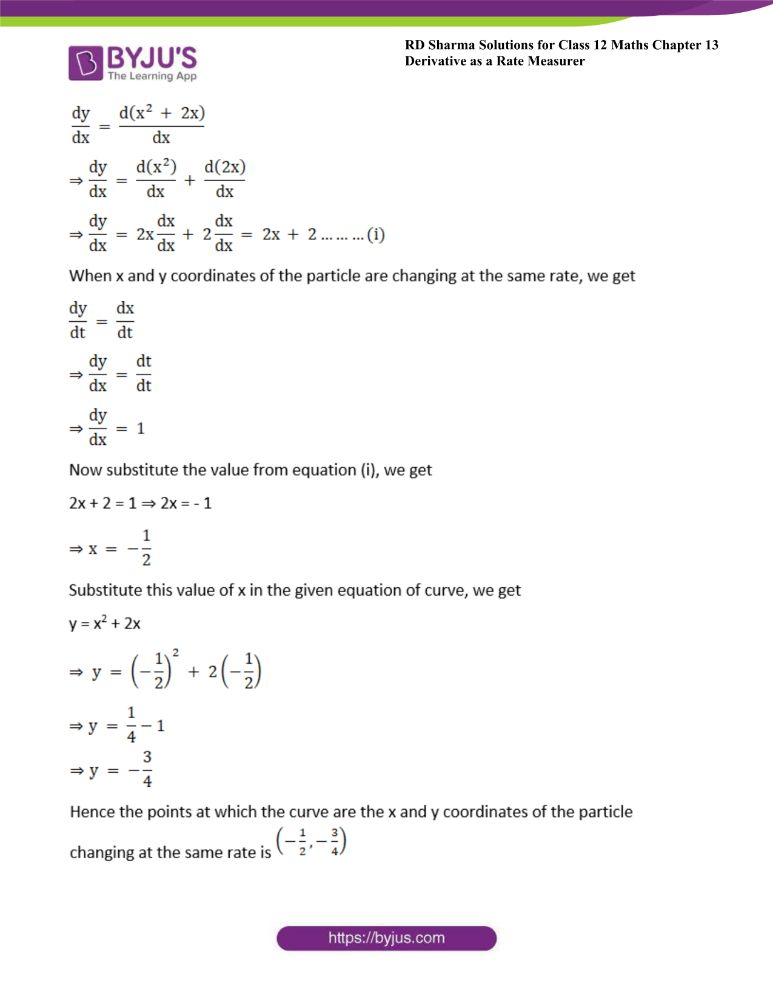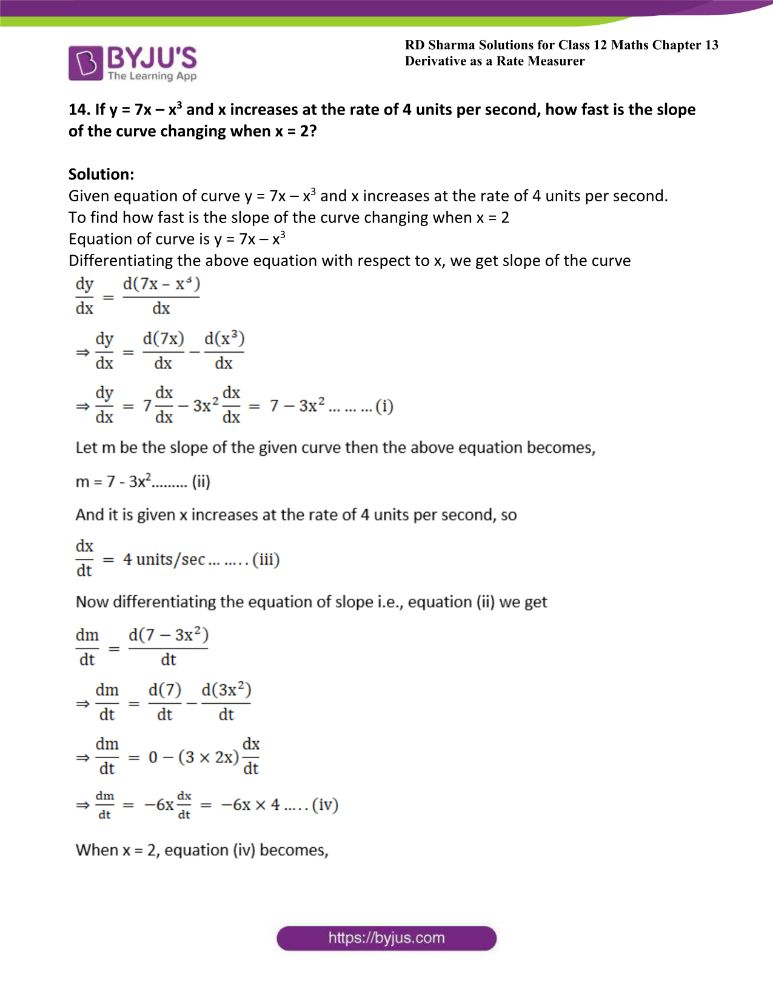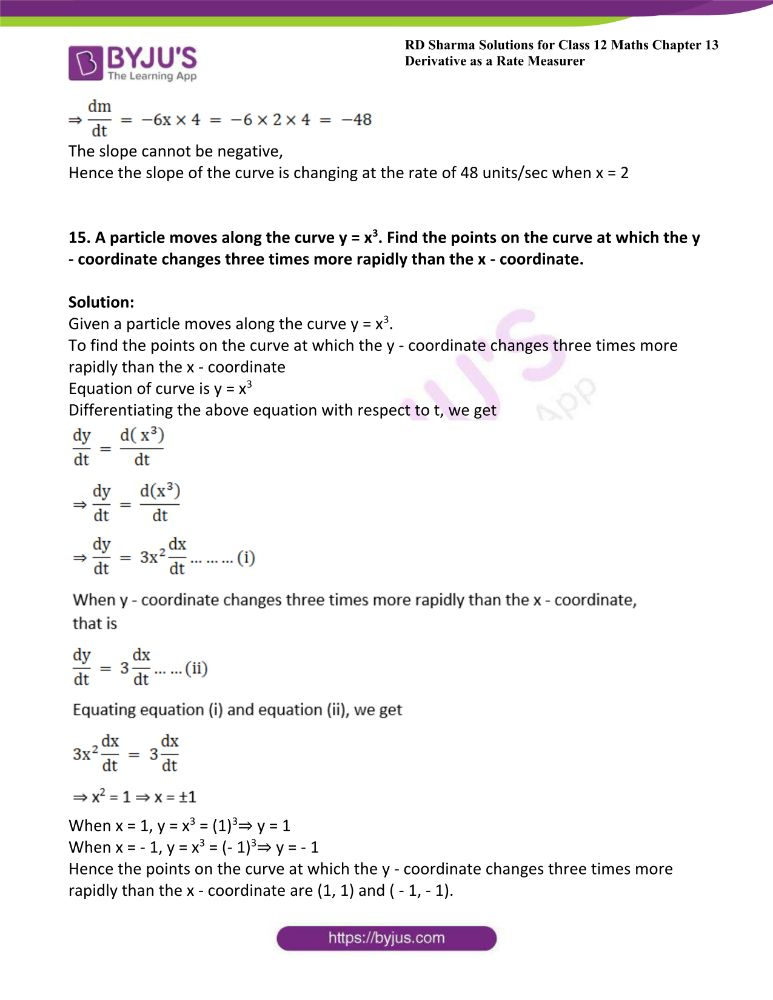### Access answers to Maths RD Sharma Solutions For Class 12 Chapter 13 – Derivatives as a Rate Measurer

Exercise 13.1 Page No: 13.4

1. Find the rate of change of the total surface area of a cylinder of radius r and height h, when the radius varies.

Solution: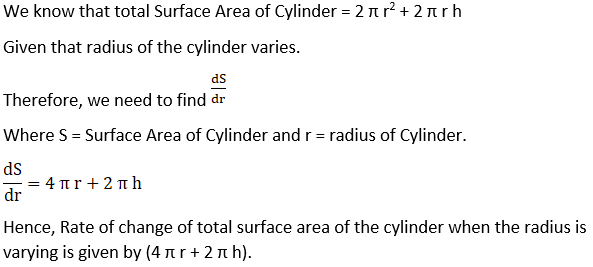2. Find the rate of change of the volume of a sphere with respect to its diameter.

Solution: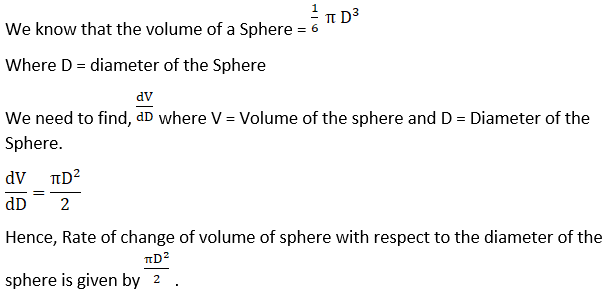3. Find the rate of change of the volume of a sphere with respect to its surface area when the radius is 2 cm.

Solution: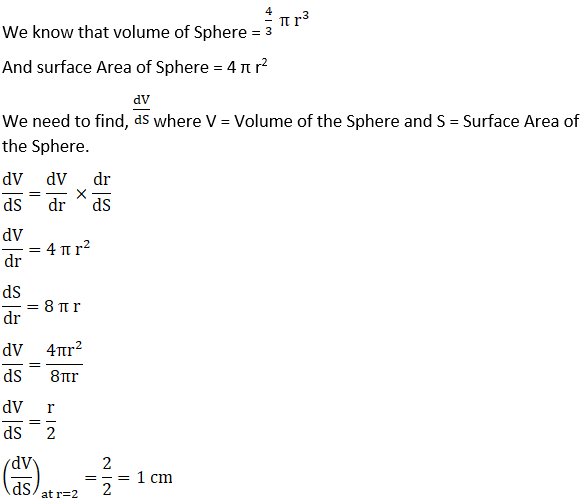4. Find the rate of change of the area of a circular disc with respect to its circumference when the radius is 3 cm.

Solution: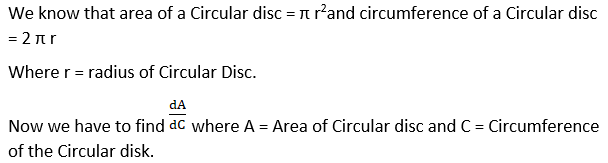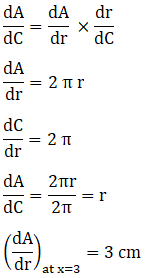5. Find the rate of change of the volume of a cone with respect to the radius of its base.

Solution: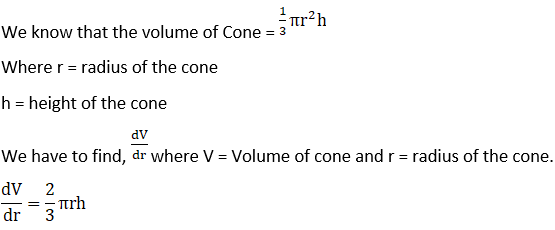Exercise 13.2 Page No: 13.19

1. The side of a square sheet is increasing at the rate of 4 cm per minute. At what rate is the area increasing when the side is 8 cm long?

Solution:

Given the side of a square sheet is increasing at the rate of 4 cm per minute.

To find rate of area increasing when the side is 8 cm long

Let the side of the given square sheet be x cm at any instant time.

Then according to the given question, we can write as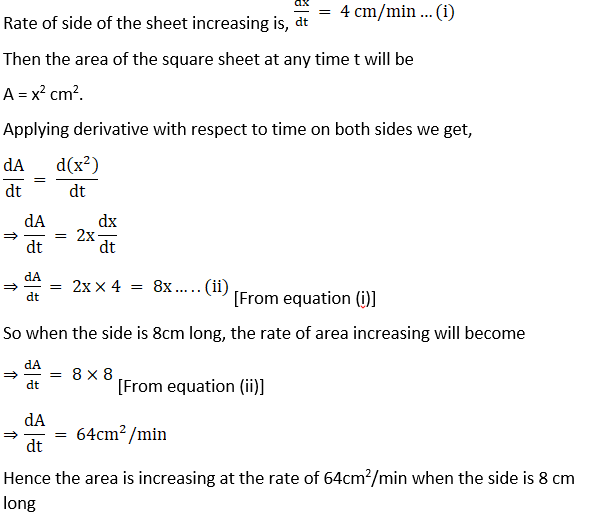2. An edge of a variable cube is increasing at the rate of 3 cm per second. How fast is the volume of the cube increasing when the edge is 1 cm long?

Solution:

Given the edge of a variable cube is increasing at the rate of 3 cm per second.

To find rate of volume of the cube increasing when the edge is 1 cm long

Let the edge of the given cube be x cm at any instant time.

Then according to the given question we can write as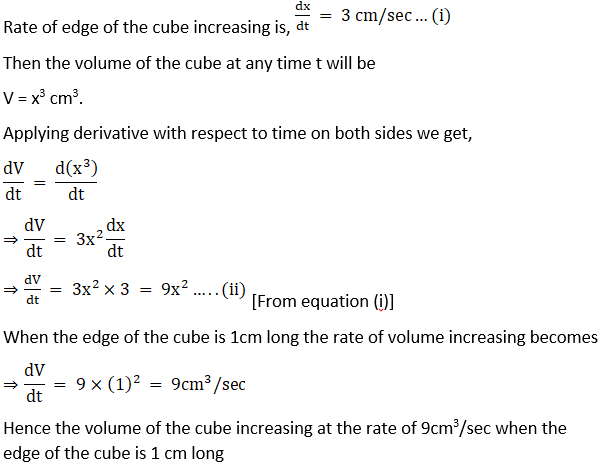3. The side of a square is increasing at the rate of 0.2 cm/sec. Find the rate of increase of the perimeter of the square.

Solution:

Given the side of a square is increasing at the rate of 0.2 cm/sec.

To find rate of increase of the perimeter of the square

Let the edge of the given cube be x cm at any instant time.

Then according to the given question, we can write as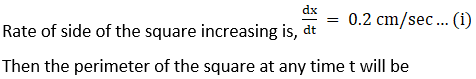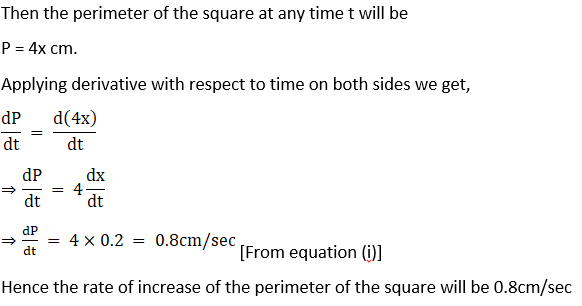4. The radius of a circle is increasing at the rate of 0.7 cm/sec. What is the rate of increase of its circumference?

Solution:

Given the radius of a circle is increasing at the rate of 0.7 cm/sec.

To find rate of increase of its circumference

Let the radius of the given circle be r cm at any instant time.

Then according to the given question, we can write as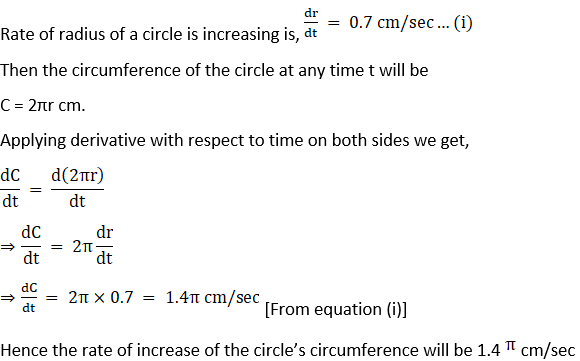Hence the rate of increase of the circle’s circumference will be 1.4 π cm/sec

5. The radius of a spherical soap bubble is increasing at the rate of 0.2 cm/sec. Find the rate of increase of its surface area, when the radius is 7 cm.

Solution:

Given the radius of a spherical soap bubble is increasing at the rate of 0.2 cm/sec.

To find rate of increase of its surface area, when the radius is 7 cm

Let the radius of the given spherical soap bubble be r cm at any instant time.

Then according to the given question we can write as

Rate of radius of the spherical soap bubble is increasing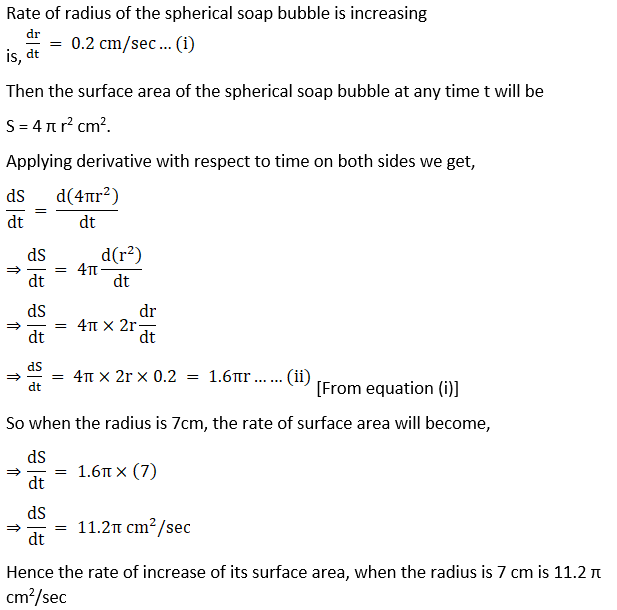Hence the rate of increase of its surface area, when the radius is 7 cm is 11.2 π cm2/sec

6. A balloon which always remains spherical is being inflated by pumping in 900 cubic centimetres of gas per second. Find the rate at which the radius of the balloon is increasing when the radius is 15 cm.

Solution:

Given spherical balloon inflated by pumping in 900 cubic centimetres of gas per second

To find the rate at which the radius of the balloon is increasing when the radius is 15 cm

Let the radius of the given spherical balloon be r cm and let V be the volume of the spherical balloon at any instant time

Then according to the given question,

As the balloon is inflated by pumping 900 cubic centimetres of gas per second hence the rate of volume of the spherical balloon increases by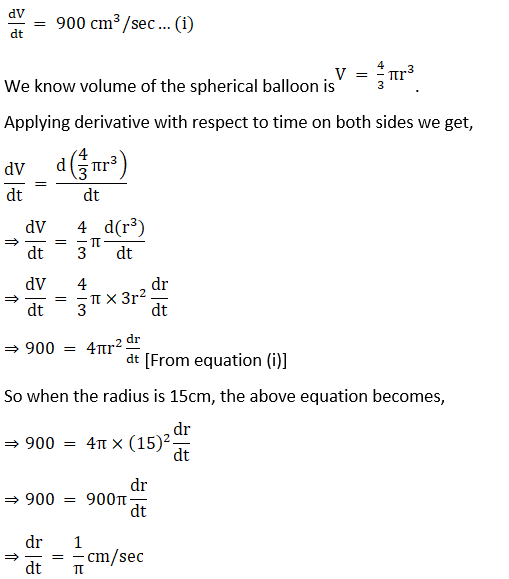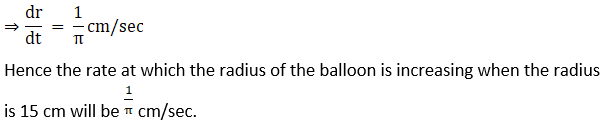7. The radius of an air bubble is increasing at the rate of 0.5 cm/sec. At what rate is the volume of the bubble increasing when the radius is 1 cm?

Solution:

Given radius of an air bubble is increasing at the rate of 0.5 cm/sec

To find the rate at which the volume of the bubble increasing when the radius is 1 cm

Let the radius of the given air bubble be r cm and let V be the volume of the air bubble at any instant time

Then according to the given question,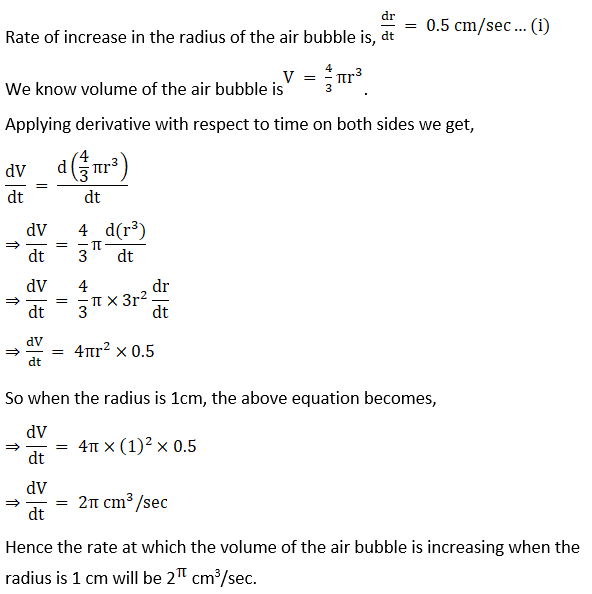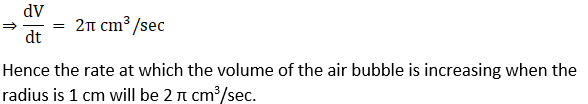8. A man 2 metres high walks at a uniform speed of 5 km/hr. away from a lamp – post 6 metres high. Find the rate at which the length of his shadow increases.

Solution:

Given a man 2 metres high walks at a uniform speed of 5 km/hr. away from a lamp – post 6 metres high

To find the rate at which the length of his shadow increases

Let AB be the lamp post and let MN be the man of height 2m.

Let AL = l meter and MS be the shadow of the man

Let length of the shadow MS = s (as shown in the below figure)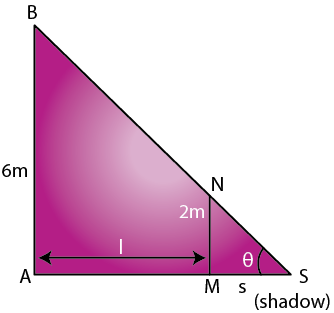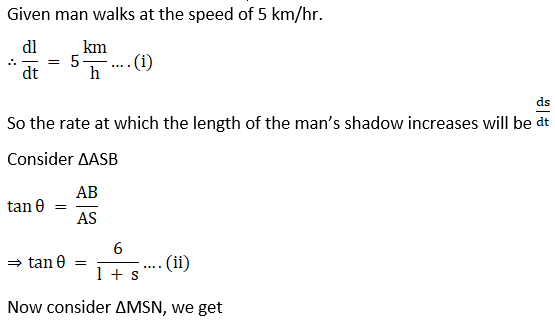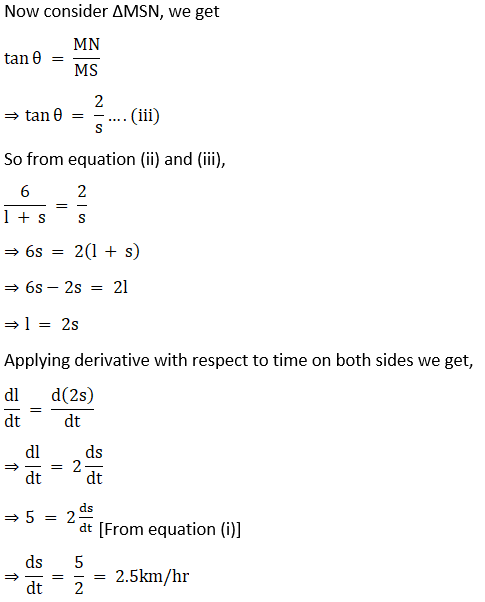Hence the rate at which the length of his shadow increases by 2.5 km/hr. and it is independent to the current distance of the man from the base of the light.

9. A stone is dropped into a quiet lake and waves move in circles at a speed of 4 cm/sec. At the instant when the radius of the circular wave is 10 cm, how fast is the enclosed area increasing?

Solution:

Given a stone is dropped into a quiet lake and waves move in circles at a speed of 4 cm/sec.

To find the instant when the radius of the circular wave is 10 cm, how fast is the enclosed area increasing

Let r be the radius of the circle and A be the area of the circle

When stone is dropped into the lake waves moves in circle at speed of 4cm/sec. i.e., radius of the circle increases at a rate of 4cm/sec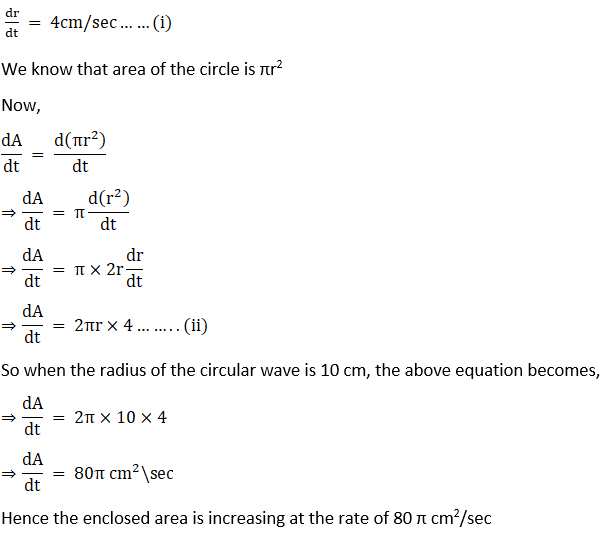10. A man 160 cm tall walks away from a source of light situated at the top of a pole 6 m high, at the rate of 1.1m/sec. How fast is the length of his shadow increasing when he is 1m away from the pole?

Solution:

Given a man 160cm tall walks away from a source of light situated at the top of a pole 6 m high, at the rate of 1.1m/sec

To find the rate at which the length of his shadow increases when he is 1m away from the pole

Let AB be the lamp post and let MN be the man of height 160cm or 1.6m.

Let AL = l meter and MS be the shadow of the man

Let length of the shadow MS = s (as shown in the below figure)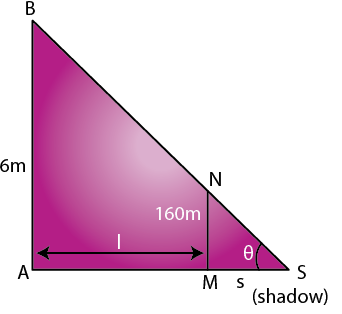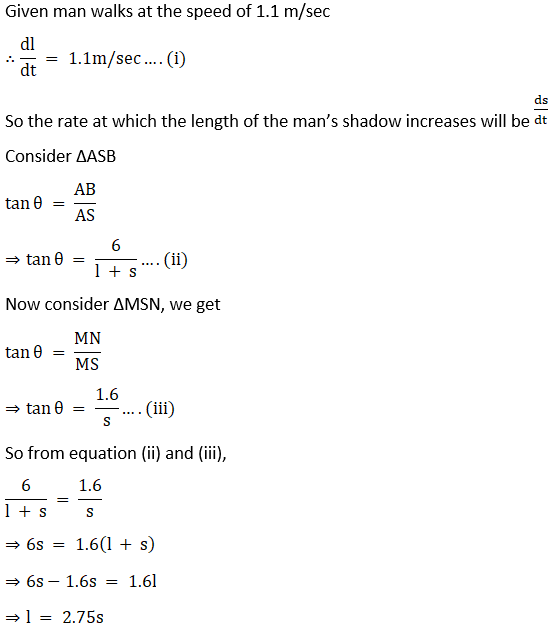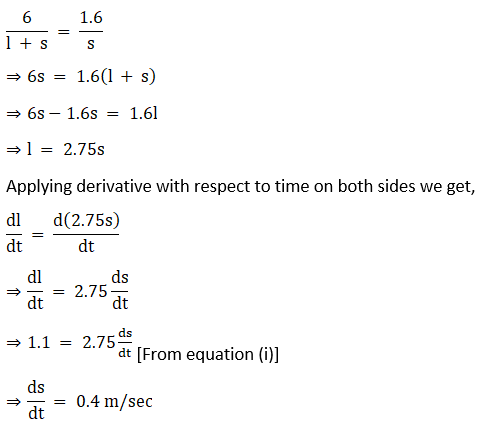Hence the rate at which the length of his shadow increases by 0.4 m/sec, and it is independent to the current distance of the man from the base of the light.

11. A man 180 cm tall walks at a rate of 2 m/sec. away, from a source of light that is 9 m above the ground. How fast is the length of his shadow increasing when he is 3 m away from the base of light?

Solution:

Given a man 180cm tall walks at a rate of 2 m/sec away; from a source of light that is 9 m above the ground

To find the rate at which the length of his shadow increases when he is 3m away from the pole

Let AB be the lamp post and let MN be the man of height 180cm or 1.8m.

Let AL = l meter and MS be the shadow of the man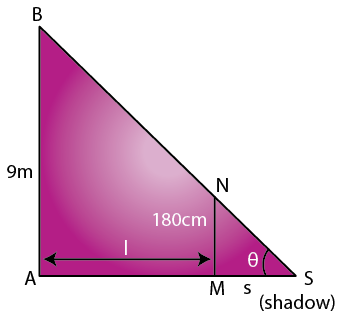Let length of the shadow MS = s (as shown in the below figure)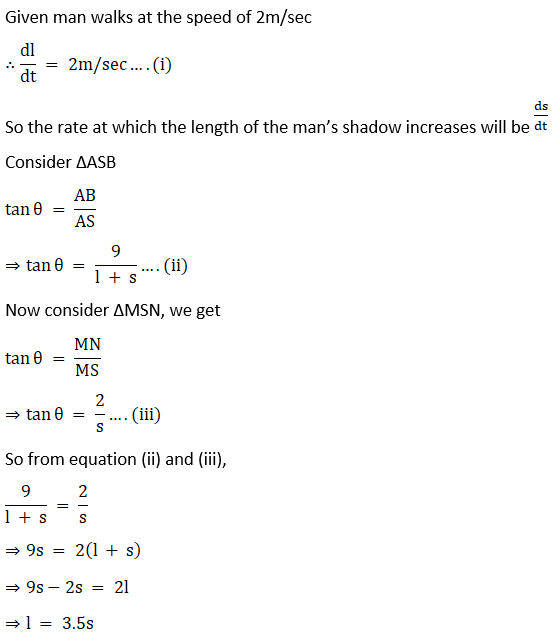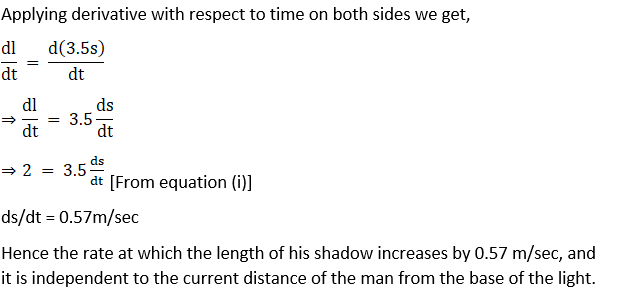ds/dt = 0.57m/sec

Hence the rate at which the length of his shadow increases by 0.57 m/sec, and it is independent to the current distance of the man from the base of the light.

12. A ladder 13 m long leans against a wall. The foot of the ladder is pulled along the ground away from the wall, at the rate of 1.5m/sec. How fast the angle θ between the ladder and the ground is is changing when the foot of the ladder is 12 m away from the wall.

Solution:

Given a ladder 13 m long leans against a wall. The foot of the ladder is pulled along the ground away from the wall, at the rate of 1.5m/sec

To find how fast is the angle θ between the ladder and the ground is changing when the foot of the ladder is 12 m away from the wall

Let AC be the position of the ladder initially, then AC = 13m.

DE be the position of the ladder after being pulled at the rate of 1.5m/sec, then DE = 13m as shown in the below figure.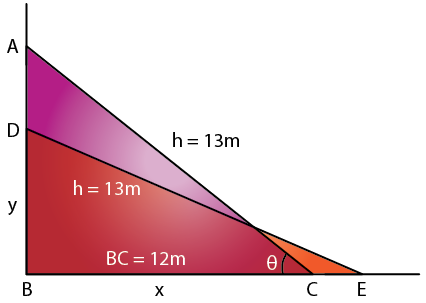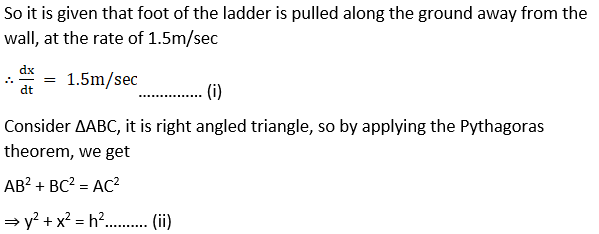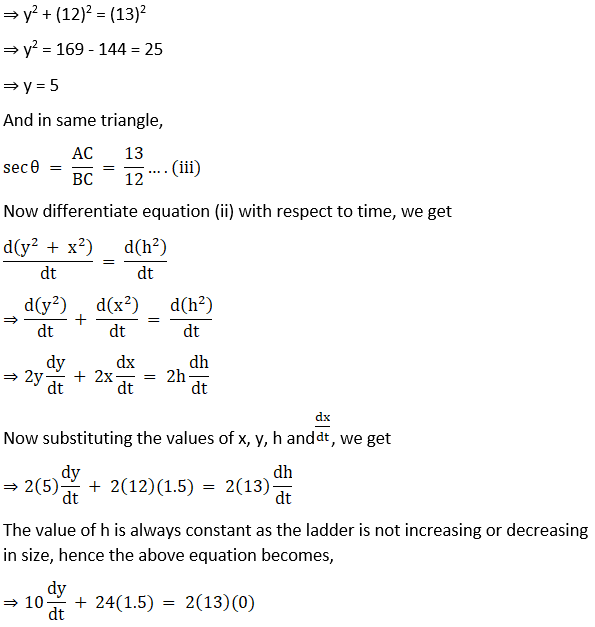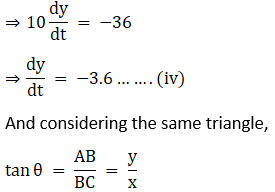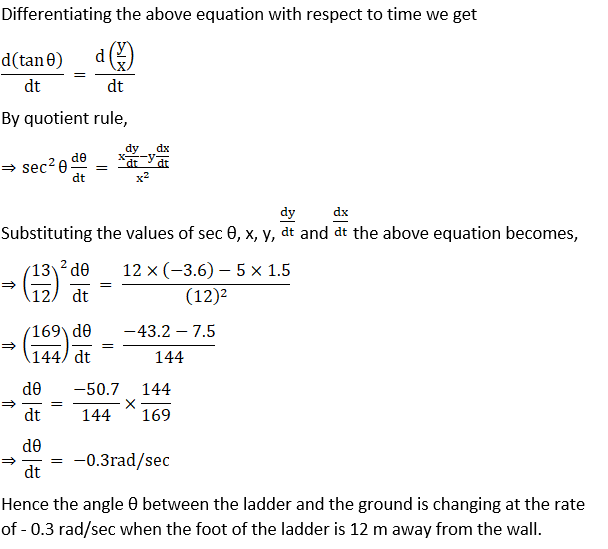Hence the angle θ between the ladder and the ground is changing at the rate of 0.3 rad/sec (because angle cannot be negative) when the foot of the ladder is 12 m away from the wall.

13. A particle moves along the curve y = x2 + 2x. At what point(s) on the curve are the x and y coordinates of the particle changing at the same rate?

Solution:

Given a particle moves along the curve y = x2 + 2x.

To find the points at which the curve are the x and y coordinates of the particle changing at the same rate

Equation of curve is y = x2 + 2x

Differentiating the above equation with respect to x, we get

dy/dx = [d(x2 + 2x)]/dx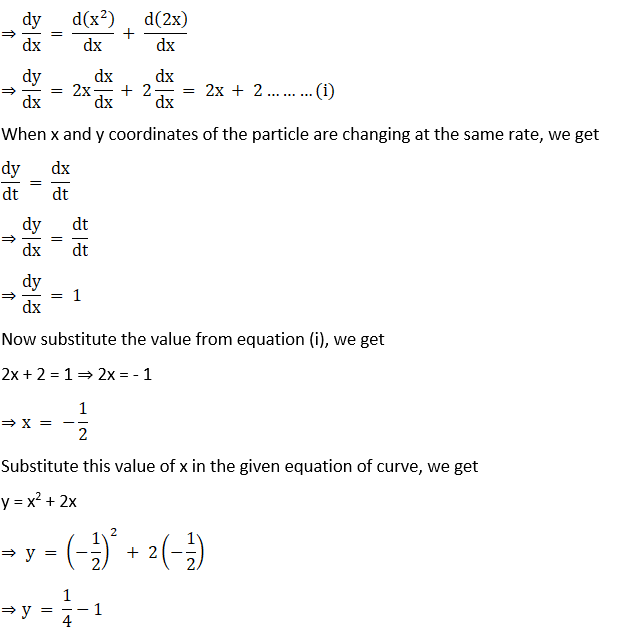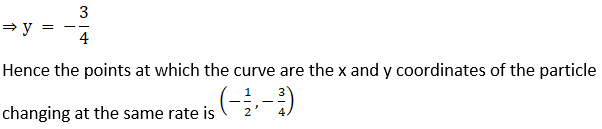14. If y = 7x – x3 and x increases at the rate of 4 units per second, how fast is the slope of the curve changing when x = 2?

Solution:

Given equation of curve y = 7x – x3 and x increases at the rate of 4 units per second.

To find how fast is the slope of the curve changing when x = 2

Equation of curve is y = 7x – x3

Differentiating the above equation with respect to x, we get slope of the curve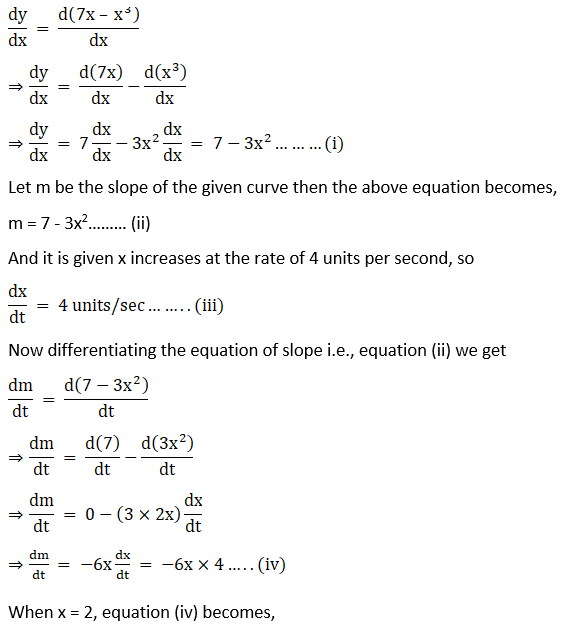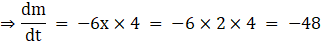The slope cannot be negative,

Hence the slope of the curve is changing at the rate of 48 units/sec when x = 2

15. A particle moves along the curve y = x3. Find the points on the curve at which the y – coordinate changes three times more rapidly than the x – coordinate.

Solution:

Given a particle moves along the curve y = x3.

To find the points on the curve at which the y – coordinate changes three times more rapidly than the x – coordinate

Equation of curve is y = x3

Differentiating the above equation with respect to t, we get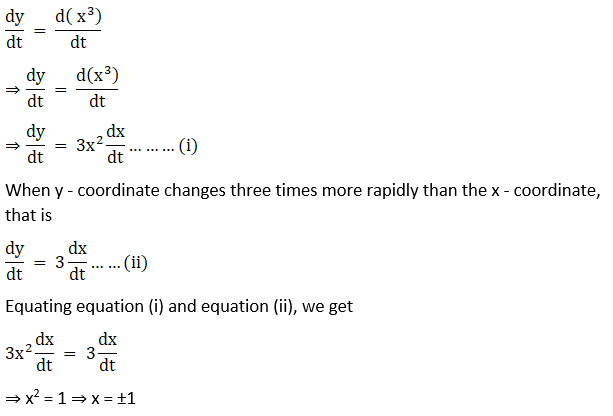When x = 1, y = x3 = (1)3⇒ y = 1

When x = – 1, y = x3 = (- 1)3⇒ y = – 1

Hence the points on the curve at which the y – coordinate changes three times more rapidly than the x – coordinate are (1, 1) and ( – 1, – 1).

### Also, access RD Sharma Solutions for Class 12 Maths Chapter 13 Derivatives as a Rate Measurer

Exercise 13.1 Solutions

Exercise 13.2 Solutions

## Frequently Asked Questions on RD Sharma Solutions for Class 12 Maths Chapter 13

### Why should one choose RD Sharma Solutions for Class 12 Maths Chapter 13 from BYJU’S?

We at BYJU’S provide 100% accurate solutions so that the students can score well in the board exams. The solutions are framed by a team of expert faculty members after conducting vast research on each concept. It mainly increases interest among students to learn new concepts without any difficulty. The simple and understandable language used in explaining the topics boost confidence among students to ace the exam without fear. Students who have doubts while answering the textbook questions can refer to the chapter solutions to get a clear idea about the overall concepts.

### Where can I get the RD Sharma Solutions for Class 12 Maths Chapter 13 online?

Students can get the RD Sharma Solutions for Class 12 Maths Chapter 13 online at BYJU’S website. Students and teachers can access BYJU’S official website for RD Sharma Solutions as well which provides a wide range of study materials like textbooks, reference books, etc. The solutions are prepared by experts with utmost care to help students understand the concepts easily and score well in their board exams.

### Does the BYJU’S RD Sharma Solutions for Class 12 Maths Chapter 13 provide accurate information for the board exam preparation?

Yes, the RD Sharma Solutions for Class 12 Maths Chapter 13 offered by BYJU’S is accurate enough based on the latest guidelines set by the CBSE board. The concepts are explained in an elaborate manner to help students grasp them without any difficulty. The expert tutors have created the solution module with utmost care so that students can learn new topics efficiently. The solutions are detailed yet easy to comprehend making it interesting for the students to refresh the concepts after learning them.

### What are the topics covered in RD Sharma Solutions for Class 12 Maths Chapter 13?

The concepts covered in RD Sharma Solutions for Class 12 Maths Chapter 13 are listed below:

• Definition of derivative as a rate measurer
• Meaning of derivative as a rate measurer
• Theorems and remarks on derivative as a rate measurer
• Definition and meaning of related rates

### Why RD Sharma Solutions for Class 12 Maths Chapter 13 are important to score good marks in board exams?

RD Sharma Solutions for Class 12 Maths Chapter 13 are curated by subject matter experts at BYJU’S as per the students grasping abilities. The solution module PDF can be accessed by students anytime and anywhere based on their requirements. All the essential concepts are explained in simple and understandable language to help students ace the exam fearlessly. Regular practice of RD Sharma Solutions designed by experts helps students clear their doubts immediately and score good marks in board exams.